# NCERT Solutions for Chapter 8 Decimals Class 6 Maths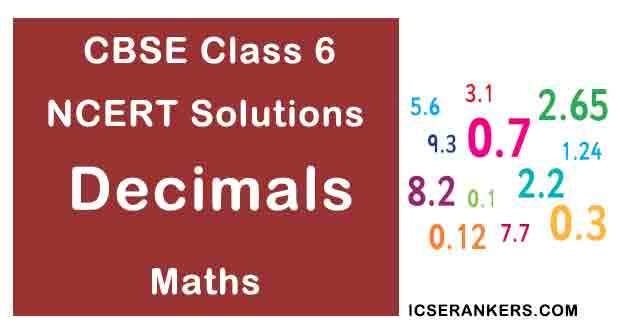Chapter Name Decimals NCERT Solutions Class CBSE Class 6 Textbook Name Maths Related Readings NCERT Solutions for Class 6NCERT Solutions for Class 6 Maths Summary of Decimals

Exercise 8.1

Question 1: Write the following as numbers in the given table: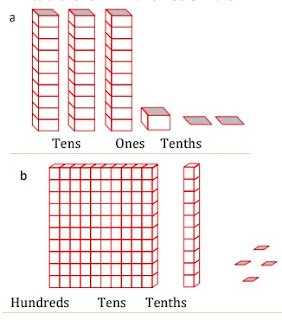Hundreds(100) Tens(10) Ones(1) Tenths(1/10)

Solution

 hundreds (100) Tens (10) Ones (1) Tenths (1/10) 0 3 2 31.2 1 1 4 110.4

Question 2: Write the following decimals in the place value table:

(a) 19.4

(b) 0.3

(c) 10.6

(d) 205.9

Solution

(a)

 hundreds Tens Ones Tenths 0 1 9 4

(b)

 hundreds Tens Ones Tenths 0 0 0 3

(c)

 hundreds Tens Ones Tenths 0 1 0 6

(d)

 hundreds Tens Ones Tenths 2 0 5 9

Question 3: Write each of the following as decimals:

(a) seven-tenths

(b) Two tens and nine-tenths

(c) Fourteen point six

(d) One hundred and two-ones

(e) Six hundred point eight

Solution

(a) seven-tenths = 7 tenths = 7/10 = 0.7

(b) 2 tens and 9-tenths = 2×10 +9/10 = 20 + 0.9 =20.9

(c) Fourteen point six = 14.6

(d) One hundred and 2-ones = 100 + 2×1 = 100 + 2 = 102

(e) Six hundred point eight = 600.8

Question 4: Write each of the following as decimals:

Solution

(a) 5/10

(b) 3+7/10

(c) 200+60++5+1/10

(d) 70+8/10

(e) 88/10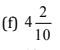(g) 3/2

(h) 2/5

(i) 12/5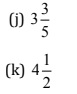Solution

(a) 5/10 = 0.5

(b) 3+7/10  = 3 + 0.7 = 3.7

(c) 200+60+5+1/10 = 200 + 60 + 5 + 0.1 = 265.1

(d) 70+8/10 = 70 + 0.8 = 70.8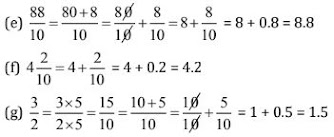(h) 2/5 = 2×2/5×2 = 4/10 = 0.4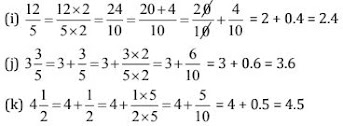Question 5: Write the following decimals as fraction. Reduce the fractions to lowest terms:

(a) 0.6

(b) 2.5

(c) 1.0

(d) 3.8

(e) 13.7

(f) 21.2

(g) 6.4

Solution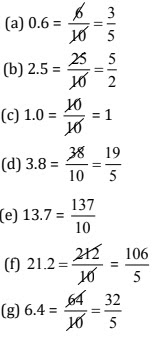Question 6: Express the following as cm using decimals:

(a) 2 mm

(b) 30 mm

(c) 116 mm

(d) 4 cm 2 mm

(e) 162 mm

(f) 83 mm

Solution

(a) ∵ 10 mm = 1 cm

∴ 1 mm = 1/10 cm

∴ 2 mm = 1/10×2 = 0.2 cm

(b) ∵ 10 mm = 1 cm

∴ 1 mm = 1/10 cm

∴ 30 mm = 1/10×30 = 3.0 cm

(c) ∵ 10 mm = 1 cm

∴ 1 mm = 1/10 cm

∴ 116 mm = 1/10×116 = 11.6 cm

(d) 4 cm+2/10 cm  [∵ 10 mm = 1cm]

4+0.2 = 4.2 cm

(e) ∵ 10 mm = 1 cm

∴ 1 mm = 1/10 cm

∴ 162 mm = 1/10×162 = 16.2 cm

(f) ∵ 10 mm = 1 cm

∴ 1 mm = 1/10 cm

∴ 83 mm = 1/10×83 = 8.3 cm

Question 7: Between which two whole numbers on the number line are the given lie? Which of these whole numbers is nearer the number?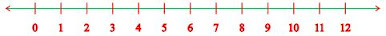(a) 0.8

(b) 5.1

(c) 2.6

(d) 6.4

(e) 9.1

(f) 4.9

Solution

(a) From 0 to 1, 0.8 is nearest to 1.

(b) From 5 to 6, 5.1 is nearest to 5.

(c) From 2 to 3, 2.6 is nearest to 3.

(d) From 6 to 7, 6.4 is nearest to 6.

(e) From 9 to 10, 9.1 is nearest to 9.

(f) From 4 to 5, 4.9 is nearest to 5.

Question 8: Show the following numbers on the number line:

(a) 0.2

(b) 1.9

(c) 1.1

(d) 2.5

Solution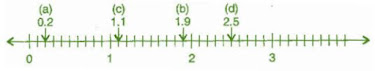Question 9: Write the decimal number represented by the points A, B, C, D on the given number line.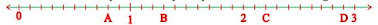Solution

A = 0 + 8/10 = 0.8

B = 1 + 3/10 = 1.3

C = 2 + 2/10 = 2.2

D = 2 + 9/10 = 2.9

Question 10: (a) The length of Ramesh’s notebook is 9 cm and 5 mm. What will be its length in cm?

(b) The length of a young gram plant is 65 mm. Express its length in cm.

Solution

(a) 9 cm 5 mm = 9 cm + 5 mm = 9 + 5/10 = 9.5 cm

(b) 65 mm = 65/10 cm = 6.5 cm

Exercise 8.2

Question 1: Complete the table with the help of these boxes and use decimals to write the number: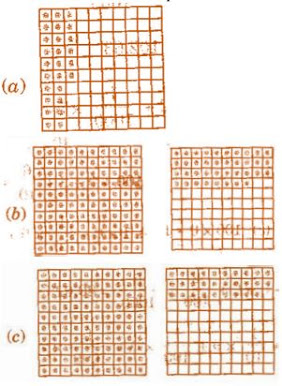Ones Tenths Hundredths Number (a) (b) (c)

Solution

 Ones Tenths Hundredths Number (a) 0 2 6 0.26 (b) 1 3 8 1.38 (c) 1 2 9 1.29

Question 2: Write the numbers given in the following place value table in decimal form:

 Hundreds (100) Tens (10) Ones (1) Tenths (1/10) Hundredths (1/100) Thousandths (1/1000) (a) 0 0 3 2 5 0 (b) 1 0 2 6 3 0 (c) 0 3 0 0 2 5 (d) 2 1 1 9 0 2 (e) 0 1 2 2 4 1

Solution

(a) 0 × 100 + 0 × 10 + 3 × 1 + 2 × 1/10 + 5 × 1/100 + 0 × 1/1000

= 0 + 0 + 3 + 0.2 + 0.05 + 0 = 3.25

(b) 1 × 100 + 0 × 10 + 2 × 1 + 6 × 1/10 + 3 × 1/100 + 0 × 1/1000

= 1 + 0 + 2 + 0.6 + 0.03 + 0 = 102.63

(c) 0 × 100 + 3 × 10 + 0 × 1 + 0 × 1/10 + 2 × 1/100 + 5 × 1/1000

= 0 + 30 + 0 + 0 + 0.02 + 0.005 = 30.025

(d) 2 × 100 + 1 × 10 + 1 × 1 + 9 × 1/10 + 0 × 1/100 + 2 × 1/1000

= 200 + 10 + 1 + 0.9 + 0 + 0.002 = 211.902

(e) 0 × 100 + 1 × 10 + 2 × 1 + 2 × 1/10 + 4 × 1/100 + 1 × 1/1000

=0 + 10 + 2 + 0.2 + 0.04 + 0.001 = 12.241

Question 3: Write the following decimals in the place value table:

(a) 0.29

(b) 2.08

(c) 19.60

(d) 148.32

(e) 200.812

Solution

 Numbers Hundreds (100) Tens (10) Ones (1) Tenths (1/10) Hundredths (1/100) Thousandths (1/1000) (a) 0.29 0 0 0 2 9 0 (b) 2.08 1 0 2 0 8 0 (c) 19.60 0 1 9 6 0 0 (d) 148.32 1 4 8 3 2 0 (e) 200.812 2 0 0 8 1 2

Question 4: Write each of the following as decimals:

(a) 20+9+4/10+1/100

(b) 137+5/100

(c) 7/10+6/100+4/1000

(d) 23+2/10+6/1000

(e) 700+20+5+9/100

Solution

(a) 20 + 9 + 0.4 + 0.01 = 29.41

(b) 137 + 0.05 = 137.05

(c) 0.7 + 0.06 + 0.004 = 0.764

(d) 23 + 0.2 + 0.006 = 23.206

(e) 700 + 20 + 5 + 0.09 = 725.09

Question 5: Write each of the following decimals in words:

(a) 0.03

(b) 1.20

(c) 108.56

(d) 10.07

(e) 0.032

(f) 5.008

Solution

(a) Zero point zero three

(b) One point two zero

(c) One hundred and eight point five six

(d) Ten point zero seven

(e) Zero point zero three two

(f) Five point zero zero eight

Question 6: Between which two numbers in tenths place on the number line does each of the given number lie?

(a) 0.06

(b) 0.45

(c) 0.19

(d) 0.66

(e) 0.92

(f) 0.57

Solution

All the numbers lie between 0 and 1.

(a) 0.06 is nearer to 0.1.

(b) 0.45 is nearer to 0.5.

(c) 0.19 is nearer to 0.2.

(d) 0.66 is nearer to 0.7.

(e) 0.92 is nearer to 0.9.

(f) 0.57 is nearer to 0.6.

Question 7: Write as fractions in lowest terms:

(a) 0.60

(b) 0.05

(c) 0.75

(d) 0.18

(e) 0.25

(f) 0.125

(g) 0.066

Solution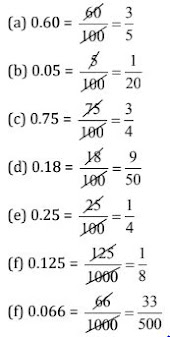Exercise 8.3

Question 1: Which is greater:

(a) 0.3 or 0.4

(b) 0.07 or 0.02

(c) 3 or 0.8

(d) 0.5 or 0.05

(e) 1.23 or 1.2

(f) 0.099 or 0.19

(g) 1.5 or 1.50

(h) 1.431 or 1.490

(i) 3.3 or 3.300

(j) 5.64 or 5.603

Solution

Before comparing, we write both terms in like decimals:

(a) 0.3 < 0.4

(b) 0.07 > 0.02

(c) 3.0 or 0.8 = 3.0 > 0.8

(d) 0.50 or 0.05 = 0.50 > 0.05

(e) 1.23 or 1.20 = 1.23 > 1.20

(f) 0.099 or 0.190 = 0.099 < 0.190

(g) 1.50 or 1.50 = 1.50 = 1.50

(h) 1.431 < 1.490

(i) 3.300 or 3.300 = 3.300 = 3.300

(j) 5.640 or 5.603 = 5.640 > 5.603

Question 2: Make five more examples and find the greater:

(a) 1.8 or 1.82

(b) 1.0009 or 1.09

(c) 10.01 or 100.1

(d) 5.100 or 5.0100

(e) 04.213 or 0421.3

Solution

Before comparing, we write both terms in like decimals

(a) 1.80 or 1.82 = 1.82 is greater than 1.8

(b) 1.0009 or 1.0900 = 1.09 is greater than 1.0009

(c) 10.01 or 100.10 = 100.1 is greater than 10.01

(d) 5.1000 or 5.0100 = 5.100 is greater than 5.0100

(e) 04.213 or 0421.300 = 0421.3 is greater than 04.213

Exercise 8.4

Question 1: Express as rupees using decimals:

(a) 5 paise

(b) 75 paise

(c) 20 paise

(d) 50 rupees 90 paise

(e) 725 paise

Solution

(a) ∵ 1 paisa = ₹ 1/100

∴ 5 paise = 1/100 × 5 = ₹ 0.05

(b) ∵ 1 paisa = ₹ 1/100

∴ 75 paise = 1/100 × 5 = ₹ 0.75

(c) ∵ 1 paisa = ₹ 1/100

∴ 20 paise = 1/100 × 5 = ₹ 0.05

(d) ∵ 1 paisa = ₹ 1/100

∴ ₹ 50+90paise=50+ 1/100 × 90 =₹50.90

(e) ∵ 1 paisa = ₹ 1/100

∴ 725 paise = 1/100 × 725 =  725/100 = ₹ 7.25

Question 2: Express as meters using decimals:

(a) 15 cm

(b) 6 cm

(c) 2 m 45 cm

(d) 9 m 7 cm

(e) 419 cm

Solution

(a) ∵ 1 cm = 1/100 m

∴ 15 cm = 1/100 × 15 = 0.15 m

(b) ∵ 1 cm = 1/100 m

∴ 6 cm = 1/100 × 6 = 0.06 m

(c) ∵ 1 cm = 1/100 m

∴ 2 m 45 cm = 2 + 1/100 × 45 = 2.45 m

(d) ∵ 1 cm = 1/100 m

∴ 9 m 7 cm = 9 + 1/100 × 7 = 9.07 m

(e) ∵ 1 cm = 1/100 m

∴ 419 cm = 1/100 × 419 = 419/100 = 4.19 m

Question 3: Express as cm using decimals:

(a) 5 mm

(b) 60 mm

(c) 164 mm

(d) 9 cm 8 mm

(e) 93 mm

Solution

(a) ∵ 1 mm = 1/10 cm

∴ 5 mm = 1/10 × 5 = 0.5 cm

(b) ∵ 1 mm = 1/10 cm

∴ 60 mm = 1/10 × 60 = 6 cm

(c) ∵ 1 mm = 1/10 cm

∴ 164 mm = 1/10 × 164 = 16.4 cm

(d) ∵ 1 mm = 1/10 cm

∴ 9cm 8mm = 9+ 1/10 × 8 =9+0.8=9.8 cm

(e) ∵ 1 mm = 1/10 cm

∴ 93 mm = 1/10 × 93 = 9.3 cm

Question 4: Express as km using decimals:

(a) 8 m

(b) 88 m

(c) 8888 m

(d) 70 km 5 m

Solution

(a) ∵ 1 m = 1/1000 km

∴ 8 m = 1/1000 × 8 = 0.008 km

(b) ∵ 1 m = 1/1000 km

∴ 88 m = 1/1000 × 88 = 0.088 km

(c) ∵ 1 m = 1/1000 km

∴ 8888 m = 1/1000 × 8888 = 8.888 km

(d) ∵ 1 m = 1/1000 km

∴ 70 km 5m =70+ 1/1000 × 5 =70.005 km

Question 5: Express as kg using decimals:

(a) 2 g

(b) 100 g

(c) 3750 g

(d) 5 kg 8 g

(e) 26 kg 50 g

Solution

(a) ∵ 1 g = 1/1000 kg

∴ 2 g = 1/1000 × 2 = 0.002 kg

(b) ∵ 1 g = 1/1000 kg

∴ 100 g = 1/1000 × 100 = 0.1 kg

(c) ∵ 1 g = 1/1000 kg

∴ 3750 g = 1/1000 × 3750 = 3.750 kg

(d) ∵ 1 g = 1/1000 kg

∴ 5 kg 8 g = 5 + 1/1000 × 8 = 5.008 kg

(e) ∵ 1 g = 1/1000 kg

∴ 26 kg 50 g = 26 + 1/1000 × 50 = 26.050 kg

Exercise 8.5

Question 1: Find the sum in each of the following:

(a) 0.007 + 8.5 + 30.08

(b) 15 + 0.632 + 13.8

(c) 27.076 + 0.55 + 0.004

(d) 25.65 + 9.005 + 3.7

(e) 0.75 + 10.425 + 2

(f) 280.69 + 25.2 + 38

Solution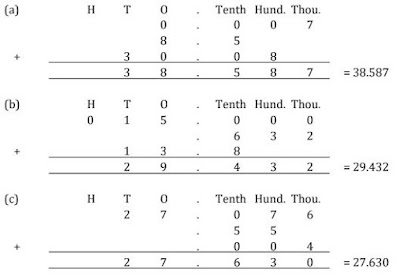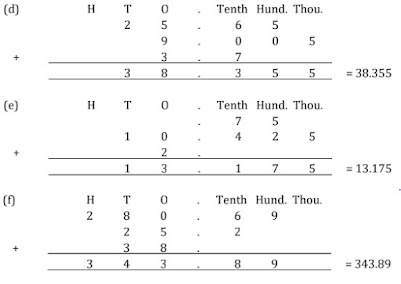Question 2: Rashid spent ₹35.75 for Maths book and ₹32.60 for Science book. Find the total amount spent by Rashid.

Solution

Money spent for Maths book = ₹35.75

Money spent for Science book = ₹32.60

Total money spent = ₹35.75 + ₹32.60 = ₹68.35

Therefore, total money spent by Rashid is ₹68.35.

Question 3: Radhika’s mother have her ₹10.50 and her father gave her ₹15.80. Find the total amount given to Radhika by the parents.

Solution

Money given by mother = ₹10.50

Money given by father = ₹15.80

Question 4: Nasreen bought 3 m 20 cm cloth for her shirt and 2 m 5 cm cloth for her trouser. Find the total length of cloth bought by her.

Solution

Cloth bought for shirt = 3 m 20 cm = 3.20 m

Cloth bought for trouser = 2 m 5 cm = 2.05 m

Total length of cloth bought by Nasreen = 3.20 + 2.05 = 5.25 m

Therefore, the total length of cloth bought by Nasreen is 5.25 m

Question 5: Naresh walked 2 km 35 m in the morning and 1 km 7 m in the evening. How much distance did he walk in all?

Solution

Distance travelled in morning = 2 km 35 m = 2.035 km

Distance travelled in evening = 1 km 7 m = 1.007 km

Total distance travelled = 2.035 + 1.007 = 3.042 km

Therefore, the total distance travelled by Naresh is 3.042 km.

Question 6: Sunita travelled 15 km 268 m by bus, 7 km 7 m by car and 500 m by foot in order to reach her school. How far is her school from her residence?

Solution

Distance travelled by bus = 15 km 268 m = 15.268 km

Distance travelled by car = 7 km 7 m = 7.007 km

Distance travelled on foot = 500 m = 0.500 km

Total distance travelled = 15.268 + 7.007 + 0.500 = 22.775 km

Therefore, total distance travelled by Sunita is 22.775 km.

Question 7: Ravi purchases 5 kg 400 g rice, 2 kg 20 g sugar and 10 kg 850 g flour. Find the total weight of his purchases.

Solution

Weight of Rice = 5 kg 400 g = 5.400 kg

Weight of Sugar = 2 kg 20 g = 2.020 kg

Weight of Flour = 10 kg 850 g = 10.850 kg

Total weight = 5.400 + 2.020 + 10.850 = 18.270 kg

Therefore, the total weight of Ravi’s purchase = 18.270 kg.

Exercise 8.6

Question 1: Subtract:

(a) ₹18.25 from ₹20.75

(b) 202.54 m from 250

(c) ₹5.36 from ₹8.40

(d) 2.051 km from 5.206 km

(e) 0.314 kg from 2.107 kg

Solution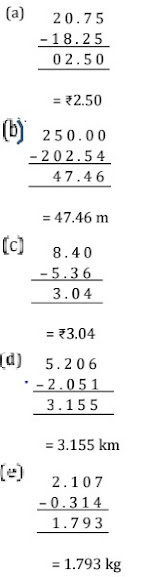Question 2: Find the value of:

(a) 9.756 – 6.28

(b) 21.05 – 15.27

(c) 18.5 – 6.79

(d) 11.6 – 9.847

Solution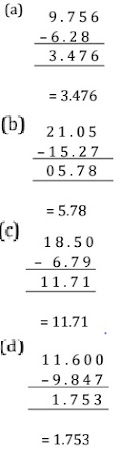Question 3: Raju bought a book of ₹35.65. He gave ₹50 to the shopkeeper. How much money did he get back from the shopkeeper?

Solution

Total amount given to shopkeeper = ₹50

Cost of book = ₹35.65

Amount left = ₹50.00 – ₹35.65

= ₹14.35

Therefore, Raju got back ₹14.35 from the shopkeeper.

Question 4: Rani had ₹18.50. She bought one ice-cream for ₹11.75. How much money does she have now?

Solution

Total money = ₹18.50

Cost of Ice-cream = ₹11.75

Amount left = ₹18.50 – ₹11.75

= ₹6.75

Therefore, Rani has ₹6.75 now.

Question 5: Tina had 20 m 5 cm long cloth. She cuts 4 m 50 cm length of cloth from this for making a curtain. How much cloth is left with her?

Solution

Total length of cloth = 20 m 5 cm = 20.05 m

Length of cloth used = 4 m 50 cm = 4.50 m

Remaining cloth = 20.05 m – 4.50 m = 15.55 m

Therefore, 15.55 m of cloth is left with Tina.

Question 6: Namita travels 20 km 50 m every day. Out of this she travels 10 km 200 m by bus and the rest by auto. How much distance does she travel by auto?

Solution

Total distance travel = 20 km 50 m = 20.050 km

Distance travelled by bus = 10 km 200 m = 10.200 km

Distance travelled by auto = 20.050 – 10.200 = 9.850 km

Therefore, 9.850 km distance travels by auto.

Question 7: Aakash bought vegetables weighing 10 kg. Out of this 3 kg 500 g in onions, 2 kg 75 g is tomatoes and the rest is potatoes. What is the weight of the potatoes?

Solution

Weight of onions = 3 kg 500 g = 3.500 kg

Weight of tomatoes = 2 kg 75 g = 2.075 kg

Total weight of onions and tomatoes = 3.500 + 2.075 = 5.575 kg

Therefore, weight of potatoes = 10.000 – 5.575 = 4.425 kg

Thus, the weight of potatoes is 4.425 kg.